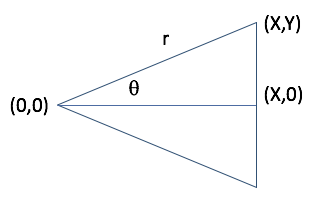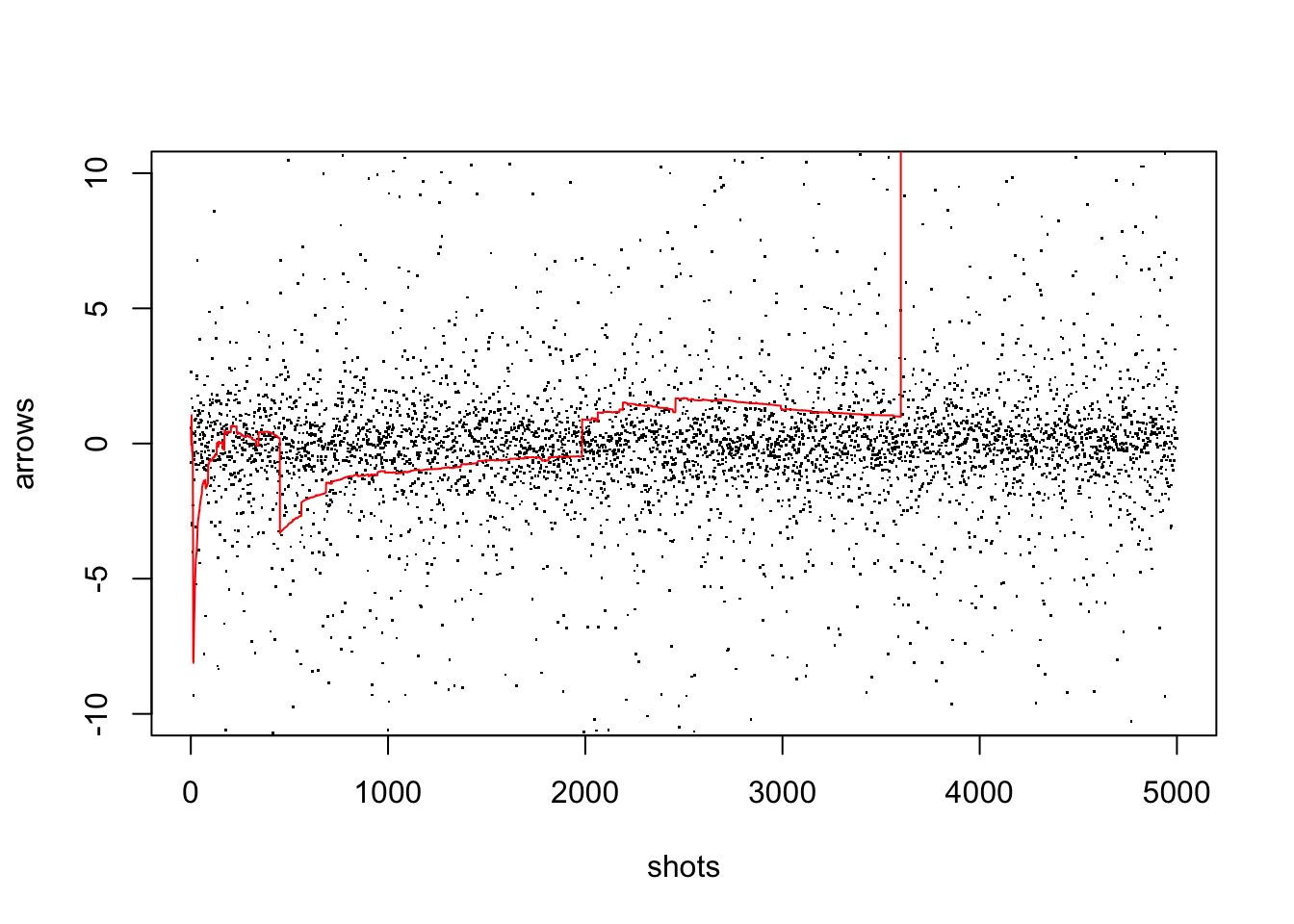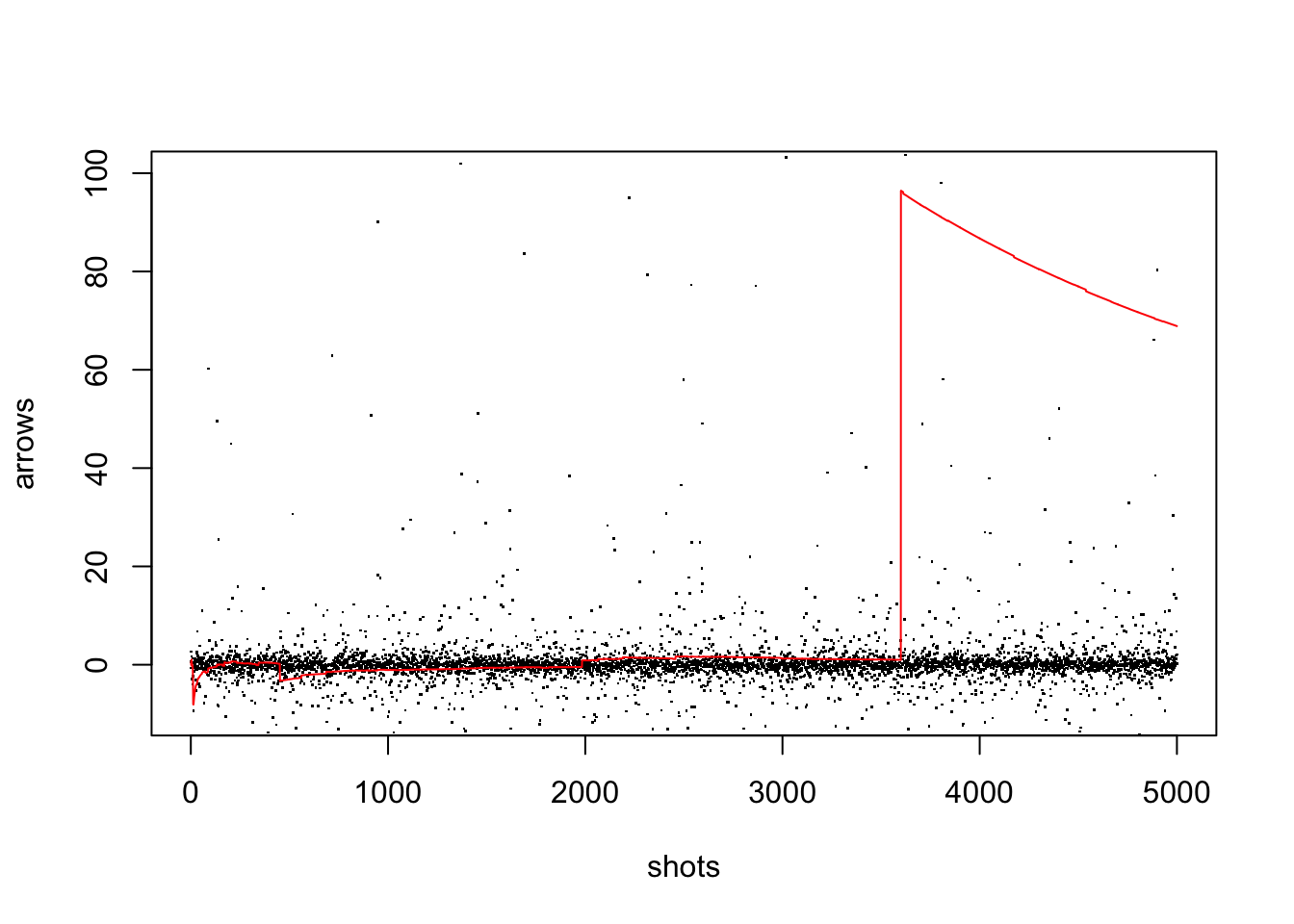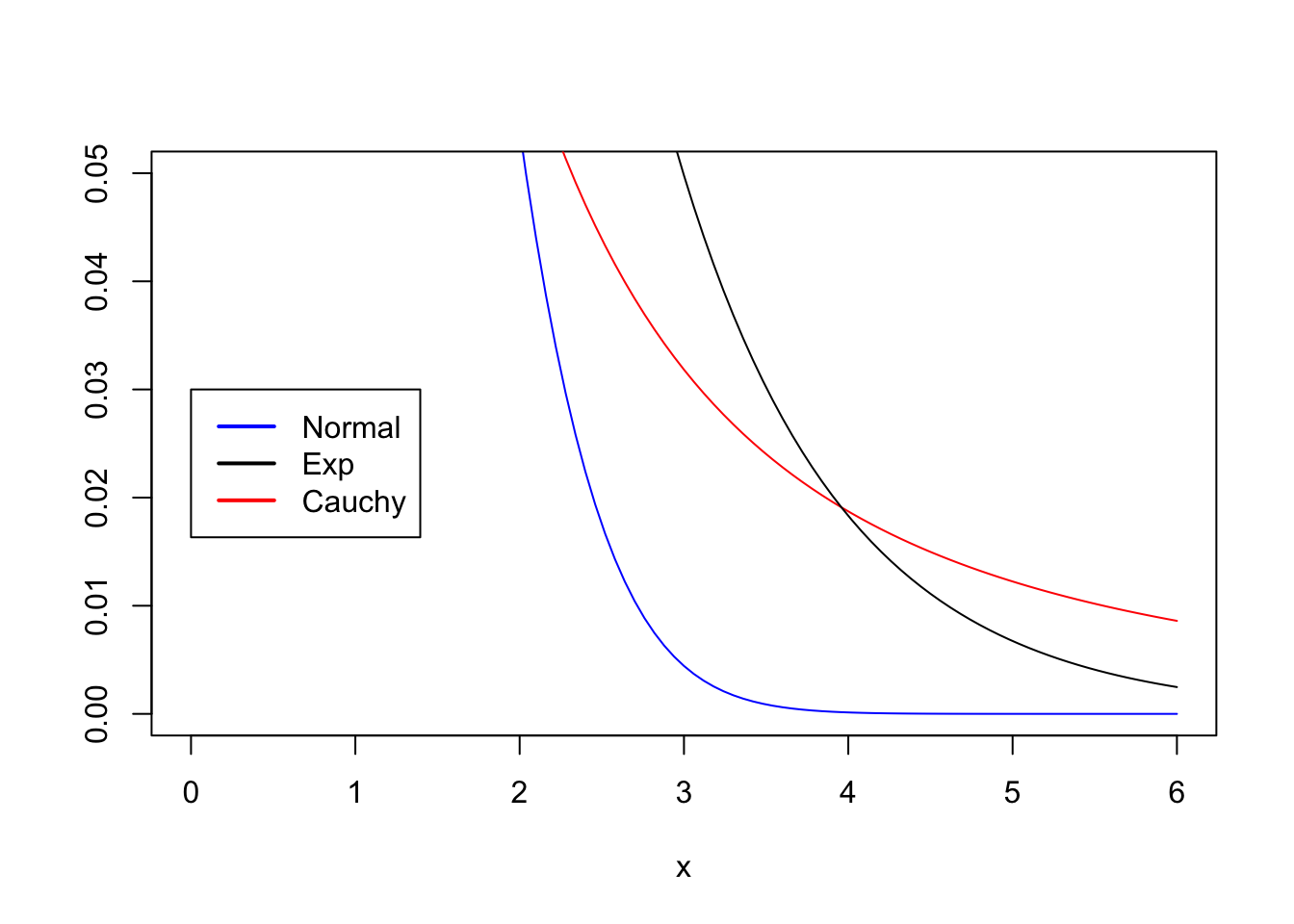# Some Notes on the Cauchy Distribution

by Joseph Rickert

by Joseph Rickert

I have always been attracted to the capricious. So, it was no surprise that I fell for the Cauchy distribution at first sight. I had never seen such unpredictability! You might say that every distribution has its moments of unpredictability, but the great charm of Cauchy is that it has no moments. (No finite moments, anyway.)

Before discussing why momentlessness (not being in the moment :) ) leads to unpredictability, let’s derive the Cauchy distribution. A common conceit for doing this is to consider a blindfolded archer trying to hit a target directly in front of him. He randomly shoots towards the wall at an angle $$\theta$$ that can sometimes be so large he shoots parallel to the wall!

Where on the wall is any given arrow likely to land? The following diagram maps out the situation.The archer is standing at the point (0, 0). The point on the wall directly in front of him is (x, 0), and the arrow will land at (x, y), (x, -y), or not at all. After changing to polar coordinates, a moments reflection will give you the equation $$y = xtan(\theta)$$.

Assuming that theta is uniformly distributed on the interval $$I = (- \pi/2, \pi/2)$$, a direct substitution into the equation for the CDF of the uniform distribution will yield the CDF for the Cauchy distribution.

$P(Y \leq y) = P(xtan(\theta) \leq y) = P(\theta \leq arctan(y/x)) = arctan(y/x) / \pi + 1/2$

Differentiating this gives the Cauchy density function: $f(y) = \frac{x}{\pi(x^{2} + y^{2})}$ This looks tame, but a short argument showing that the necessary integrals do not converge demonstrates that neither the mean nor the variance exist. Hence, neither the Law of Large Numbers, nor the Central Limit Theorem apply. Taking lots of samples and computing averages doesn’t buy you anything. The averages just don’t settle down. This behavior is apparent in the following simulation that computes means of Cauchy samples for sample sizes of one to five thousand. The plots that show the same data at different scales dramatize the erratic behavior.

set.seed(pi)
N <- 5000
shots <- 1:N
arrows <- rcauchy(N)
means <- cumsum(arrows) / shots
summary(means)
##    Min. 1st Qu.  Median    Mean 3rd Qu.    Max.
## -8.1130 -0.6115  1.1500 22.7800 71.0000 96.4300
plot(shots, arrows, pch = ".", ylim = c(-10, 10))
points(shots, means, type = "l", col = "red")plot(shots, arrows, pch = ".", ylim = c(-10, 100))
points(shots, means, type = "l", col = "red")Not only don’t the samples converge, it is not that difficult to show that the distribution of the sample mean:

$Y =\frac{Y_{1} + Y_{2} . . . + Y_{n} }{n}$ of n independent Cauchy random variables has the same distribution as a single Cauchy random variable! The proof is straightforward. Let $$\phi(t)$$ be the characteristic function. Then
$\phi_{Y}(t) = [\phi_{Y1}(t)]^n= e^{-n|t|}$ which is the characteristic function of nY.

The extreme values that dominate the Cauchy distribution make it the prototypical heavy-tailed distribution. Informally, a distribution is often described as having heavy or “fat” tails if the probability of events in the tails of the distribution are greater than what would be given by a Normal distribution. While there seems to be more than one formal definition of a [heavy-tailed distribution] (https://en.wikipedia.org/wiki/Heavy-tailed_distribution), the following diagram, which compares the right tails of the Normal, Exponential and Cauchy distributions, gets the general idea across.

# Plot right tails of distributions
low <- 0; high <- 6
curve(dnorm,from = low, to = high, ylim = c(0, .05), col = "blue", ylab = " ", add = FALSE)
curve(dcauchy,from = low, to = high, col = "red", add = TRUE)
curve(dexp,from = low, to = high, col = "black", add = TRUE)

legend(0,0.03, c("Normal", "Exp", "Cauchy"),
lty=c(1,1,1), # symbols (lines)
lwd=c(2,2,2), col=c("blue", "black", "red"))As exotic as the Cauchy distribution may seem, it is not all that difficult to come face-to-face with the Cauchy Distribution in every-day modeling work. A student t distribution with one degree of freedom is Cauchy, as is the ratio of two independent standard normal random variables.

Additionally, the Cauchy distribution, also called the Breit-Wigner, or Lorentz distribution, has applications in particle physics, spectroscopy, finance, and medicine. In his 2006 JSS paper, Geroge Marsaglia elaborates on early work he did on transforming the ratio of two jointly Normal random variables into something tractable. The original problem arose from an attempt to estimate the intercept in a linear model giving the life span of red blood cells.

The real fun, and maybe the real world, seems to happen when things are not normal.